Apples 2

How many apples were originally on the tree, if the first day fell one third, the second day quarter of the rest and on tree remained 45 apples?

Result

x =  90

Solution:

x/3 + (x-x/3)/4+45 = x

6x = 540

x = 90

Calculated by our simple equation calculator.

Leave us a comment of this math problem and its solution (i.e. if it is still somewhat unclear...):Be the first to comment!To solve this verbal math problem are needed these knowledge from mathematics:

Do you have a linear equation or system of equations and looking for its solution? Or do you have quadratic equation?

Next similar math problems:

1. FactoryIn the factory workers work in three shifts. In the first inning operates half of all employees in the second inning and a third in the third inning 200 employees. How many employees work at the factory?
2. Stamps and albumsMichail has in three albums stored 170 stamps. In the first album, there are 14 more than in the second and in the second is by 1/5 less than in the third. How many stamps are in the first album?
3. Influenza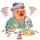For the first week of February by influenza 5% of children in kindergarten. The second week ill 9 next children, bringing the number of patients increased to 20%. How many children went to the kindergarten?
4. Mushrooms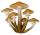For five days, we have collected 410 mushrooms. Interestingly every day we have collected 10 mushrooms more than the preceding day. How many mushrooms we have collected during 4th day?
5. CandyPeter had a sachet of candy. He wanted to share with his friends. If he gave them 30 candies, he would have 62 candies. If he gave them 40 candies, he would miss 8 candies. How many friends did Peter have?
6. Mom and daughterMother is 39 years old. Her daughter is 15 years. For many years will mother be four times older than the daughter?
7. FriendsJames and Jaroslav are friends. Together they had 48 toy cars. But if John took 8 cars from Jaroslav, Jaroslav will be have 1.5 times more toy cars than James. How many toy cars had Jaroslav and James?
8. Ski class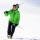Class attend 30 boys and some number of girls. Ski training was attended by 28 boys and all the girls, which was 95% of all students. How many girls attend this class? How many percent is that?
9. TestingStudents of high school have 10 points for each good solved task. The wrong answer is deducted by 5 points. After solving 20 tasks, student Michael had 80 points. How many tasks did he solve correctly and how many wrong?
10. Ski lessons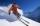On the ski course leaves 80 pupils. They live in triple and quadruple rooms. Count if rooms is the 23. How many are triple and quadruple rooms?
11. Three workersThree workers were rewarded CZK 9200 and the money divided by the work they have done. First worker to get twice than the second, the second three times more than the third. How much money each worker received?
12. Walnutsx walnuts were in the mission. Dano took 1/4 of nuts Michael took 1/8 from the rest and John took 34 nuts. It stayed here 29 nuts. Determine the original number of nuts.
13. Unknown numberIdentify unknown number which 1/5 is 40 greater than one tenth of that number.
14. Simple equationSolve for x: 3(x + 2) = x - 18
15. Fifth of the numberThe fifth of the number is by 24 less than that number. What is the number?
16. EquationSolve the equation: 1/2-2/8 = 1/10; Write the result as a decimal number.
17. Percentage reduction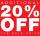Reducing the unknown number by 19% get number 700.7 Determine unknown number.